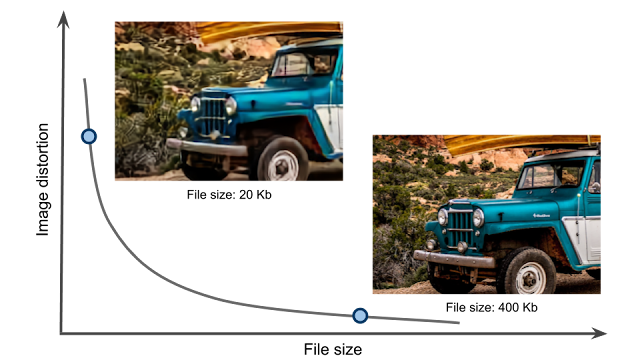# Optimizing Multiple Loss Functions with Loss-Conditional Training

In many machine learning applications the performance of a model cannot be summarized by a single number, but instead relies on several qualities, some of which may even be mutually exclusive. For example, a learned image compression model should minimize the compressed image size while maximizing its quality. It is often not possible to simultaneously optimize all the values of interest, either because they are fundamentally in conflict, like the image quality and the compression ratio in the example above, or simply due to the limited model capacity. Hence, in practice one has to decide how to balance the values of interest.The trade-off between the image quality and the file size in image compression. Ideally both the image distortion and the file size would be minimized, but these two objectives are fundamentally in conflict.
The standard approach to training a model that must balance different properties is to minimize a loss function that is the weighted sum of the terms measuring those properties. For instance, in the case of image compression, the loss function would include two terms, corresponding to the image reconstruction quality and the compression rate. Depending on the coefficients on these terms, training with this loss function results in a model producing image reconstructions that are either more compact or of higher quality.

If one needs to cover different trade-offs between model qualities (e.g. image quality vs compression rate), the standard practice is to train several separate models with different coefficients in the loss function of each. This requires keeping around multiple models both during training and inference, which is very inefficient. However, all of these separate models solve very related problems, suggesting that some information could be shared between them.

In two concurrent papers accepted at ICLR 2020, we propose a simple and broadly applicable approach that avoids the inefficiency of training multiple models for different loss trade-offs and instead uses a single model that covers all of them. In “You Only Train Once: Loss-Conditional Training of Deep Networks”, we give a general formulation of the method and apply it to several tasks, including variational autoencoders and image compression, while in “Adjustable Real-time Style Transfer”, we dive deeper into the application of the method to style transfer.

Loss-Conditional Training
The idea behind our approach is to train a single model that covers all choices of coefficients of the loss terms, instead of training a model for each set of coefficients. We achieve this by (i) training the model on a distribution of losses instead of a single loss function, and (ii) conditioning the model outputs on the vector of coefficients of the loss terms. This way, at inference time the conditioning vector can be varied, allowing us to traverse the space of models corresponding to loss functions with different coefficients.

This training procedure is illustrated in the diagram below for the style transfer task. For each training example, first the loss coefficients are randomly sampled. Then they are used both to condition the main network via the conditioning network and to compute the loss. The whole system is trained jointly end-to-end, i.e., the model parameters are trained concurrently with random sampling of loss functions.Overview of the method, using stylization as an example. The main stylization network is conditioned on randomly sampled coefficients of the loss function and is trained on a distribution of loss functions, thus learning to model the entire family of loss functions.
The conceptual simplicity of this approach makes it applicable to many problem domains, with only minimal changes to existing code bases. Here we focus on two such applications, image compression and style transfer.

Application: Variable-Rate Image Compression
As a first example application of our approach, we show the results for learned image compression. When compressing an image, a user should be able to choose the desired trade-off between the image quality and the compression rate. Classic image compression algorithms are designed to allow for this choice. Yet, many leading learned compression methods require training a separate model for each such trade-off, which is computationally expensive both at training and at inference time. For problems such as this, where one needs a set of models optimized for different losses, our method offers a simple way to avoid inefficiency and cover all trade-offs with a single model.

We apply the loss-conditional training technique to the learned image compression model of Balle et al. The loss function here consists of two terms, a reconstruction term responsible for the image quality and a compactness term responsible for the compression rate. As illustrated below, our technique allows training a single model covering a wide range of quality-compression tradeoffs.Compression at different quality levels with a single model. All animations are generated with a single model by varying the conditioning value.Adjustable style transfer. All stylizations are generated with a single network by varying the conditioning values.